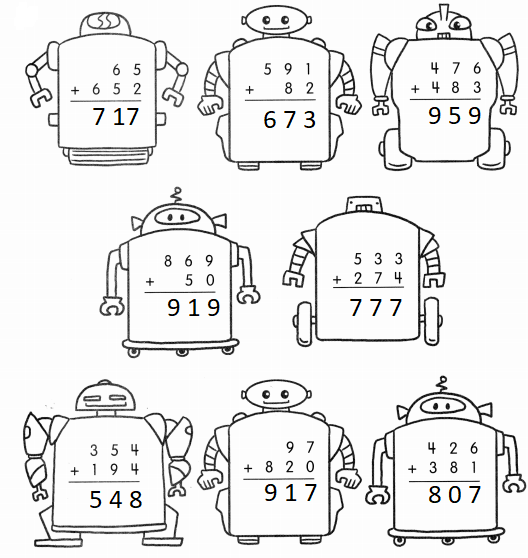# Math in Focus Grade 2 Chapter 2 Practice 5 Answer Key Addition with Regrouping in Tens

Go through the Math in Focus Grade 2 Workbook Answer Key Chapter 2 Practice 5 Addition with Regrouping in Tens to finish your assignments.

## Math in Focus Grade 2 Chapter 2 Practice 5 Answer Key Addition with Regrouping in Tens

Question 1.
534 + 283 = ?
4 ones + 3 ones = ones
3 tens + 8 tens = ___ tens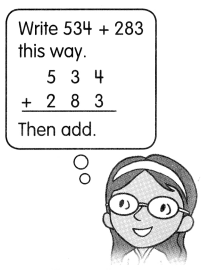= ___ hundred ___ ten
1 hundred + 5 hundreds + 2 hundreds = ___ hundreds
534 + 283 = ____
534 + 283 = 817
Explanation:
4 ones + 3 ones = 7 ones
3 tens + 8 tens = 11 tens
= 1 hundred 1 ten
1 hundred + 5 hundreds + 2 hundreds = 8 hundreds

Question 2.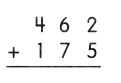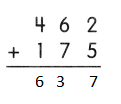Explanation:
2  ones + 5 ones = 7 ones
6 tens + 7 tens = 13 tens
= 1 hundred 3 ten
1 hundred + 4 hundreds + 1 hundreds = 6 hundreds

Question 3.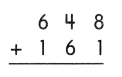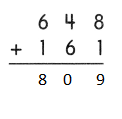Explanation:
8 ones + 1 ones = 9 ones
4  tens + 6 tens = 10 tens
= 1 hundred 0 ten
1 hundred + 6 hundreds + 1 hundreds = 8 hundreds

Question 4.
295 + 633 = ___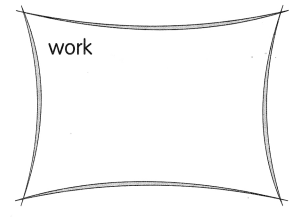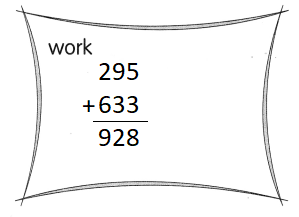Explanation:
5 ones + 3 ones = 8 ones
9 tens + 3  tens = 12 tens
= 1 hundred 2 ten
1 hundred + 2 hundreds + 6 hundreds = 9 hundreds

Question 5.
462 + 456 = ___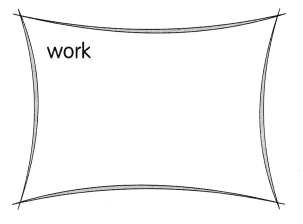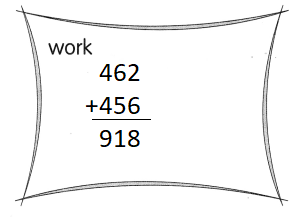Explanation:
2 ones + 6 ones = 8 ones
6 tens + 5 tens = 11 tens
= 1 hundred 1 ten
1 hundred + 4 hundreds + 4 hundreds = 9 hundreds